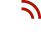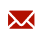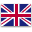Staxx Pallet Truck is a professional warehouse equipment manufacturer and pallet jack supplier in China.+86-574-89217230  |info@cnmhe.comEnglish

# Graphical Method of Forklift Angle Error

by：Staxx Pallet Truck     2021-06-24
The angle error analysis is an indispensable work in the design of steering mechanism. A small angle error can improve the transmission efficiency of the mechanism, realize the approximate concentric rolling of the inner and outer steering wheels during the steering process, and reduce the wear of the wheels and tires. At present, the steering mechanism of counterbalanced forklifts (hereinafter referred to as forklifts) mostly adopts through-type transverse cylinders. The analysis of the angle error is generally divided into calculation method or drawing method. In the calculation method, the calculation of the actual angle of the formula is more complicated, and manual work is required. The graph error is too large to grasp accurate data. This article introduces the mapping method of using CAXA software for corner analysis. The general specific steps are as follows: 　　1. First draw the geometric model of the steering trapezoid and the theoretical characteristic curves of the front and rear axes and the inner and outer corners. As shown in Figure 1, polygon OABCDO1 is a schematic diagram of the steering mechanism of a forklift, which is symmetrical along the longitudinal axis of the forklift, where O and O1 are the turning centers of the left and right steering wheels, AB and CD are connecting rods, and BC is the distance between the connecting holes of the horizontal cylinder. O. Circle O1 is the trajectory circle of the connecting hole of the steering knuckle arm, EF is the center line of the front axle, and EG is the theoretical characteristic line of the rotation angle. 2. Take the horizontal stroke of the cylinder S (Su003d58 in Figure 1) to intercept the point on BC with B as the starting point, take the intercept point as the center, and AB length (Ru003d110 in Figure 1) as the radius on the circle O Obtain the intersection point. The line connecting this point and the center O and OA form an angle (56.1° in Figure 1), which is the maximum outer rotation angle. Use CAXA's 'straight line-angle division line' command to divide the angle into several equal parts. In this example, 10 equal divisions are used. In practical applications, the angle is divided as much as possible, and the bisecting line forms an intersection with the circle O.　　3. Select the angle dividing line and boundary line made in the previous step, and use the 'rotate-start and end point-copy' command to set the group of lines with O as the base point, point A as the starting point, and the intersection of circle O and OO1 Rotate the copy counterclockwise for the end point, and then use the 'same edge' method to make the group of lines intersect with the EG line. Starting from point A, take the intersection of the angle bisector and the boundary line at the circle O as the starting point, and draw the arcs in sequence with the AB length as the radius, so that each arc and BC form an intersection.　　4. Use 'translation-copy' to move the above arcs along the BC direction (463 in Figure 1) and copy them, and form an intersection with the BC extension line, starting from point C, with point C and each intersection as the center of the circle, Use CD as the radius to make arcs in sequence, so that each arc and circle O1 have intersection points, and connect each intersection point and circle center O1 to form a set of fan-shaped straight lines. This is the actual inner corner line and the included angle between the two boundary fan-shaped straight lines (see figure The 79.3° angle in 1) is the maximum internal rotation angle. 5. Copy the above-mentioned actual inner corner line group with the OO1 line as the symmetry axis mirror direction, as shown in Figure 2, mirror point D to point H, use the 'rotate-start and end point-rotate' command to this group of lines Take O1 as the base point, H point as the starting point, and the intersection of circle O1 and OO1 as the ending point to rotate counterclockwise (rotation copy is used to show the process in Figure 2), and use the 'Single edge' command to make the straight line and the EG line, outside The corner bisector forms the point of intersection.　　 6. Use the spline curve to connect the intersection of the inner and outer corner lines in turn to form the curve near EG in Figure 1, which is the actual corner characteristic curve. Connect O1 and the intersection formed by the outer corner bisector and the EG line. In order to facilitate the distinction, this group of lines can be set as layer lines with different colors and linear shapes. These lines are the theoretical inner corner lines. In the design of electric forklifts, in order to facilitate production organization, a steering axle is often used on several products with close specifications and different wheelbases. At this time, the front axis and EF line in the above figure are theoretically deleted from the inner corner line. Re-draw, and make the outer corner bisector intersect with EF and draw a new theoretical inner corner line. Only need to repeat the seventh step of the above process, you can easily get the steering angle error of the steering mechanism under different wheelbases. For example, when the steering axle is used in a forklift with a wheelbase of 1350 in this example, the maximum turning angle error value is 3.21° after drawing, which appears when the outer turning angle is 44.9° and the inner turning angle is 59.9°. Therefore, this method is especially useful in practical applications. The serialized design is extremely convenient and fast, and it is also relatively accurate.
At a time when technology is essential for pallet stacker truck, ensuring that it works in a symbiotic way with your human employees is key.
If you are ready to stop the problem of semi electric pallet stacker and go back to normal, contact us at Staxx Pallet Stackers. Ningbo Staxx Material Handling Equipment Co.,Ltd. is ready to help you out.
This is crucial when you need to maintain innovative information in pallet stacker truck.
Time is one of the biggest challenges cited by manufacturing pallet stacker truck.
We utilize our expertise to develop services that add value at each phase of thepallet stacker truck development cycle. We evaluate and implement new strategies in response to changing customer profiles and market conditions.
Custom message

Chat Online 编辑模式下无法使用